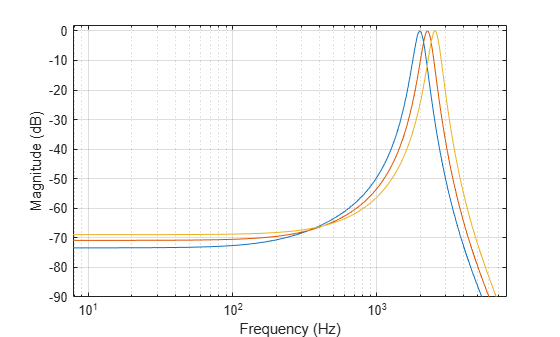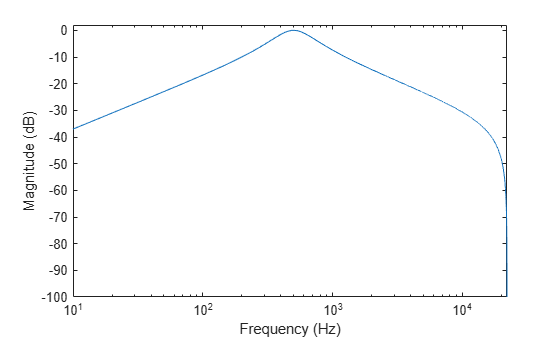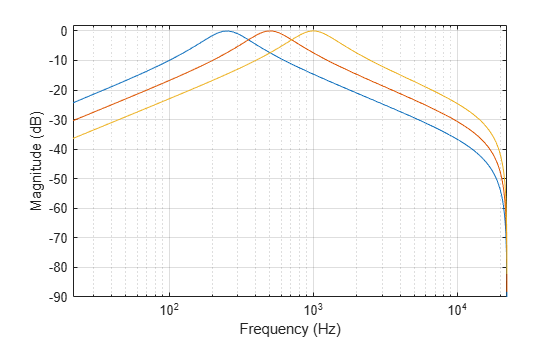# freqz

Compute frequency response

## Syntax

``[H,f] = freqz(obj)``
``[H,f] = freqz(obj,ind)``
``[H,f] = freqz(___,Name,Value)``
``freqz(___)``

## Description

example

````[H,f] = freqz(obj)` returns a matrix of complex frequency responses for each filter designed by `obj`.```

example

````[H,f] = freqz(obj,ind)` returns the frequency response of filters with indices corresponding to the elements in vector `ind`.```

example

````[H,f] = freqz(___,Name,Value)` specifies options using one or more `Name,Value` pair arguments.```

example

````freqz(___)` with no output arguments plots the frequency response of the filter bank.```

## Examples

collapse all

Create a `gammatoneFilterBank` object. Call `freqz` to get the complex frequency response, `H`, of the filter bank and a vector of frequencies, `f`, at which the response is calculated. Plot the magnitude frequency response of the filter bank.

```gammaFiltBank = gammatoneFilterBank; [H,f] = freqz(gammaFiltBank); plot(f,abs(H)) xlabel('Frequency (Hz)')```To get the frequency response of a subset of filters in the filter bank, specify the second argument as a row vector of indices between one and the number of filters in the filter bank. Get the frequency response of the 10th filter in the filter bank and plot the magnitude frequency response.

```[H,f] = freqz(gammaFiltBank,10); plot(f,abs(H)) xlabel('Frequency (Hz)')```To specify the number of FFT points used to compute the frequency response, use the `NFFT` name-value pair. Specify that the frequency response is calculated using a 128-point FFT. Plot the magnitude frequency response.

```[H,f] = freqz(gammaFiltBank,'NFFT',128); plot(f,abs(H)) xlabel('Frequency (Hz)')```To visualize the magnitude frequency response only, call `freqz` without any output arguments. Plot the magnitude frequency response, in dB, of filters 20, 21, and 22 using a 1024-point DFT.

`freqz(gammaFiltBank,[20,21,22],'NFFT',1024)`Create an `octaveFilterBank` object. Call `freqz` to get the complex frequency response, `H`, of the filter bank and a vector of frequencies, `f`, at which the response is calculated. Plot the magnitude frequency response in dB.

```octFiltBank = octaveFilterBank; [H,f] = freqz(octFiltBank); plot(f,20*log10(abs(H))) xlabel('Frequency (Hz)') ylabel('Magnitude (dB)') set(gca,'XScale','log') axis([10 octFiltBank.SampleRate/2 -100 2])```To get the frequency response of a subset of filters in the filter bank, specify the second argument as a row vector of indices between one and the number of filters in the filter bank. Get the frequency response of the 5th filter in the filter bank and plot the magnitude frequency response in dB.

```[H,f] = freqz(octFiltBank,5); plot(f,20*log10(abs(H))) xlabel('Frequency (Hz)') ylabel('Magnitude (dB)') set(gca,'XScale','log') axis([10 octFiltBank.SampleRate/2 -100 2])```To specify the number of FFT points used to compute the frequency response, use the `NFFT` name-value pair. Specify that the frequency response is calculated using a 8192-point FFT. Plot the magnitude frequency response in dB.

```[H,f] = freqz(octFiltBank,'NFFT',8192); plot(f,20*log10(abs(H))) xlabel('Frequency (Hz)') ylabel('Magnitude (dB)') set(gca,'XScale','log') axis([10 octFiltBank.SampleRate/2 -100 2])```To visualize the magnitude frequency response only, call `freqz` without any output arguments. Plot the magnitude frequency response, in dB, of filters 4, 5, and 6 using a 1024-point DFT.

`freqz(octFiltBank,[4,5,6],'NFFT',1024)`## Input Arguments

collapse all

Object to get filter frequency responses from, specified as an object of `gammatoneFilterBank` or `octaveFilterBank`.

Indices of filters to calculate frequency responses from, specified as a row vector of integers with values in the range [1, N]. N is the total number of filters designed by `obj`.

### Name-Value Arguments

Specify optional pairs of arguments as `Name1=Value1,...,NameN=ValueN`, where `Name` is the argument name and `Value` is the corresponding value. Name-value arguments must appear after other arguments, but the order of the pairs does not matter.

Before R2021a, use commas to separate each name and value, and enclose `Name` in quotes.

Example: `'NFFT',2048`

Number of DFT bins, specified as a positive integer.

Data Types: `single` | `double` | `int8` | `int16` | `int32` | `int64` | `uint8` | `uint16` | `uint32` | `uint64`

## Output Arguments

collapse all

Complex frequency response of each filter, returned as an M-by-N matrix. M is the number of DFT bins, specified by `NFFT`. N is the number of filters, which is either `length(ind)` or, if `ind` is not specified, the total number of filters in the filter bank.

Data Types: `double`

Frequencies at which the response is computed in Hz, returned as a column vector.

Data Types: `double`

## Version History

Introduced in R2019a